# 遗传算法—genetic algorithms

Genetic programming is a branch of genetic algorithms. The main difference between genetic programming and genetic algorithms is the representation of the solution. Genetic programming creates computer programs in the lisp or scheme computer languages as the solution. Genetic algorithms create a string of numbers that represent the solution. Genetic programming, uses four steps to solve problems:
1) Generate an initial population of random compositions of the functions and terminals of the problem (computer programs).

2) Execute each program in the population and assign it a fitness value according to how well it solves the problem.

3) Create a new population of computer programs.
i) Copy the best existing programs
ii) Create new computer programs by mutation.
iii) Create new computer programs by crossover(sexual reproduction).

4) The best computer program that appeared in any generation, the best-so-far solution, is designated as the result of genetic programming [Koza 1992].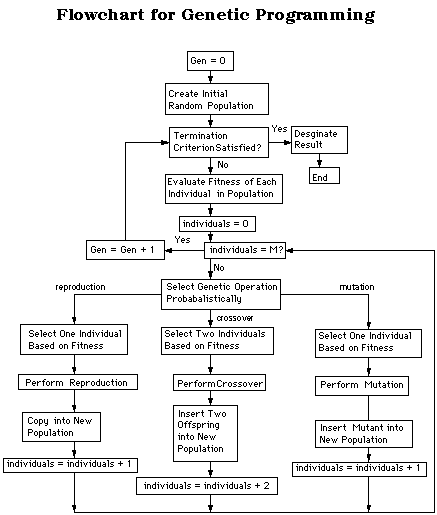Fitness Function
The most difficult and most important concept of genetic programming is the fitness function. The fitness function determines how well a program is able to solve the problem. It varies greatly from one type of program to the next. For example, if one were to create a genetic program to set the time of a clock, the fitness function would simply be the amount of time that the clock is wrong. Unfortunately, few problems have such an easy fitness function; most cases require a slight modification of the problem in order to find the fitness.

Gun Firing Program
A more complicated example consists of training a genetic program to fire a gun to hit a moving target. The fitness function is the distance that the bullet is off from the target. The program has to learn to take into account a number of variables, such as wind velocity, type of gun used, distance to the target, height of the target, velocity and acceleration of the target. This problem represents the type of problem for which genetic programs are best. It is a simple fitness function with a large number of variables.

Water Sprinkler System
Consider a program to control the flow of water through a system of water sprinklers. The fitness function is the correct amount of water evenly distributed over the surface. Unfortunately, there is no one variable encompassing this measurement. Thus, the problem must be modified to find a numerical fitness. One possible solution is placing water-collecting measuring devices at certain intervals on the surface. The fitness could then be the standard deviation in water level from all the measuring devices. Another possible fitness measure could be the difference between the lowest measured water level and the ideal amount of water; however, this number would not account in any way the water marks at other measuring devices, which may not be at the ideal mark.

Maze Solving Program
If one were to create a program to find the solution to a maze, first, the program would have to be trained with several known mazes. The ideal solution from the start to finish of the maze would be described by a path of dots. The fitness in this case would be the number of dots the program is able to find. In order to prevent the program from wandering around the maze too long, a time limit is implemented along with the fitness function.

Functions and Terminals
The terminal and function sets are also important components of genetic programming. The terminal and function sets are the alphabet of the programs to be made. The terminal set consists of the variables and constants of the programs. In the maze example, the terminal set would contain three commands: forward, right and left. The function set consists of the functions of the program. In the maze example the function set would contain: If "dot" then do x else do y. In the gun firing program is the terminal set would be composed of the different variables of the problem. Some of these variables could be the velocities and accelerations of the gun, the bullet and target. The functions are several mathematical functions, such as addition, subtraction, division, multiplication and other more complex functions.

Crossover Operation
Two primary operations exist for modifying structures in genetic programming. The most important one is the crossover operation. In the crossover operation, two solutions are sexually combined to form two new solutions or offspring. The parents are chosen from the population by a function of the fitness of the solutions. Three methods exist for selecting the solutions for the crossover operation.

The first method uses probability based on the fitness of the solution. If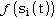is the fitness of the solution Si and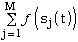is the total sum of all the members of the population, then the probability that the solution Si will be copied to the next generation is [Koza 1992]: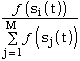Another method for selecting the solution to be copied is tournament selection. Typically the genetic program chooses two solutions random. The solution with the higher fitness will win. This method simulates biological mating patterns in which, two members of the same sex compete to mate with a third one of a different sex. Finally, the third method is done by rank. In rank selection, selection is based on the rank, (not the numerical value) of the fitness values of the solutions of the population [Koza 1992].

The creation of the offsprings from the crossover operation is accomplish by deleting the crossover fragment of the first parent and then inserting the crossover fragment of the second parent. The second offspring is produced in a symmetric manner. For example consider the two S-expressions in Figure 2, written in a modified scheme programming language and represented in a tree.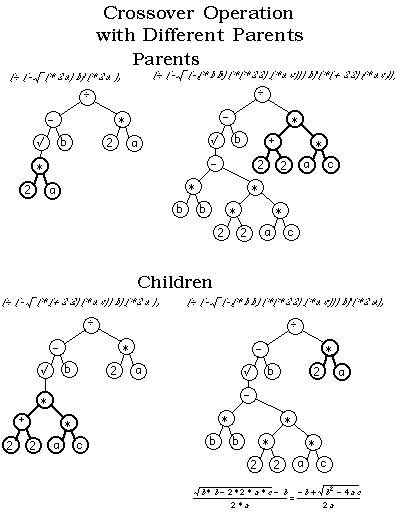Figure 2: Crossover operation for genetic programming. The bold selections on both parents are swapped to create the offspring or children. (The child on the right is the parse tree representation for the quadratic equation.)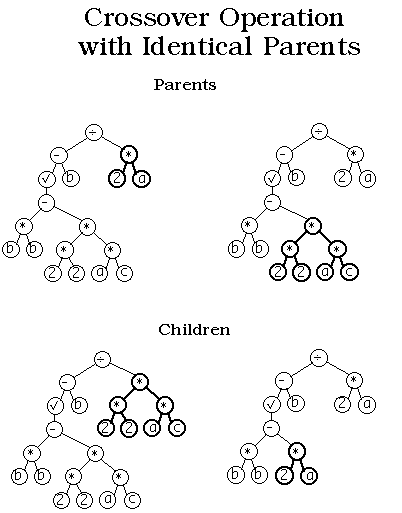Figure 3: This figure illustrates one of the main advantages of genetic programming over genetic algorithms. In genetic programming identical parents can yield different offspring, while in genetic algorithms identical parents would yield identical offspring. The bold selections indicate the subtrees to be swapped.

An important improvement that genetic programming displays over genetic algorithms is its ability to create two new solutions from the same solution. In the Figure 3 the same parent is used twice to create two new children.

Mutation
Mutation is another important feature of genetic programming. Two types of mutations are possible. In the first kind a function can only replace a function or a terminal can only replace a terminal. In the second kind an entire subtree can replace another subtree. Figure 4 explains the concept of mutation: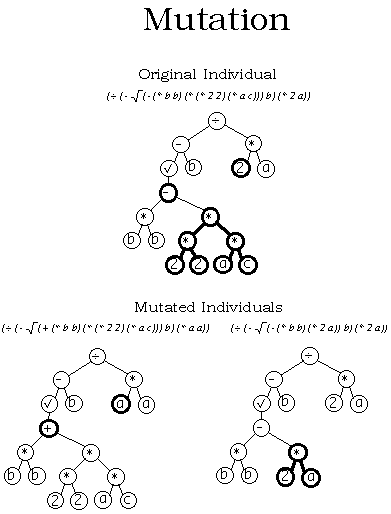Figure 4: Two different types of mutations. The top parse tree is the original agent. The bottom left parse tree illustrates a mutation of a single terminal (2) for another single terminal (a). It also illustrates a mutation of a single function (-) for another single function (+). The parse tree on the bottom right illustrates a the replacement of a subtree by another subtree.

Summary
Genetic programming is much more powerful than genetic algorithms. The output of the genetic algorithm is a quantity, while the output of the genetic programming is a another computer program. In essence, this is the beginning of computer programs that program themselves.

Genetic programming works best for several types of problems. The first type is where there is no ideal solution, (for example, a program that drives a car). There is no one solution to driving a car. Some solutions drive safely at the expense of time, while others drive fast at a high safety risk. Therefore, driving a car consists of making compromises of speed versus safety, as well as many other variables. In this case genetic programming will find a solution that attempts to compromise and be the most efficient solution from a large list of variables.

Furthermore, genetic programming is useful in finding solutions where the variables are constantly changing. In the previous car example, the program will find one solution for a smooth concrete highway, while it will find a totally different solution for a rough unpaved road.

REFERENCES

Koza, John R. 1992. Genetic Programming: On the Programming of Computers by Means of Natural Selection. Cambridge, MA: The MIT Press.

Cramer, Nichael Lynn: "A Representation for the Adaptive Generation of Simple Sequential Programs", Proceedings, International Conference on Genetic Algorithms and their Applications, July 1985 [CMU], pp183-187.

01-099141
06-233万+
12-059898
05-173703
03-081612
06-223171
07-213601
10-23277
07-241202
07-08773
03-035250
09-146673
09-111487

### “相关推荐”对你有帮助么？

•非常没帮助
•没帮助
•一般
•有帮助
•非常有帮助被折叠的  条评论 为什么被折叠?到【灌水乐园】发言点击重新获取扫码支付1.余额是钱包充值的虚拟货币，按照1:1的比例进行支付金额的抵扣。
2.余额无法直接购买下载，可以购买VIP、付费专栏及课程。余额充值